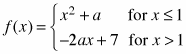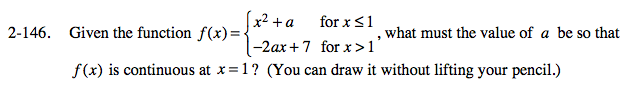### Home > PC > Chapter 2 > Lesson 2.3.6 > Problem2-146

2-146.

Given the function, what must the value of a be so that f(x) is continuous at x = 1? (You can draw it without lifting your pencil.) Try this using the 2-146 eTool (Desmos). Homework Help ✎Visualize this set of functions using the eTool below.

Set the two parts of the function equal to each other for the value of x = 1.

x2 + 1 = −2ax + 7 for x = 1

Substitute 1 in for x and solve for a.